# Revision Notes For CBSE Class 10 Maths Chapter 8 Introduction to Trigonometry

In chapter 8, students will mainly learn about two topics which are trigonometric ratios and trigonometric identities. Students will further study how to calculate trigonometric ratios for specific and complementary angles and how to establish identities for the ratios. While this is the main objective of the chapter, students can download and make use of the CBSE revision notes for class 10 maths chapter 8 – introduction to trigonometry to increase their understanding of all the concepts present in this chapter. The notes contain clear and crisp information and can be used as effective study materials. Once students get familiar with the notes they will be able to develop better math fluency and solve even difficult questions without any hassle.

Class 10 maths chapter 8 CBSE revision notes PDF can be accessed below.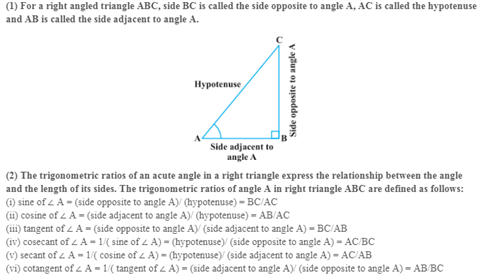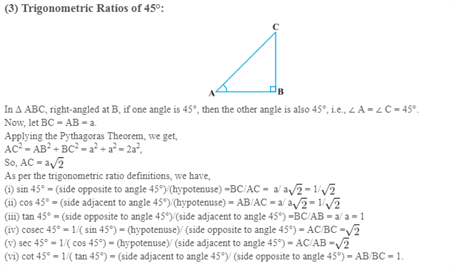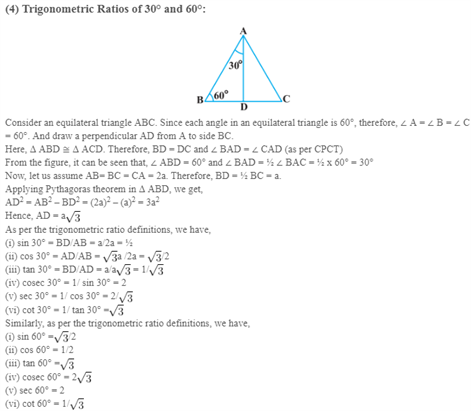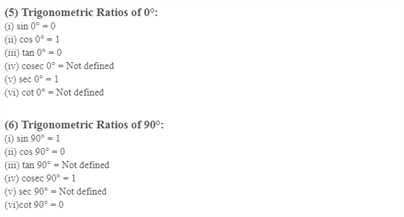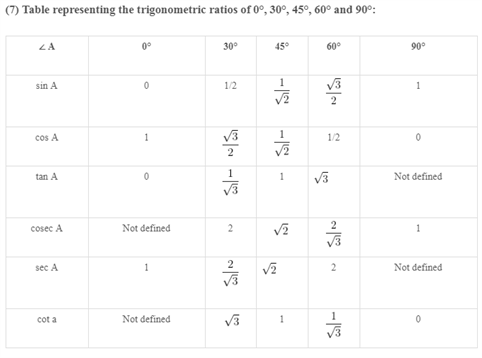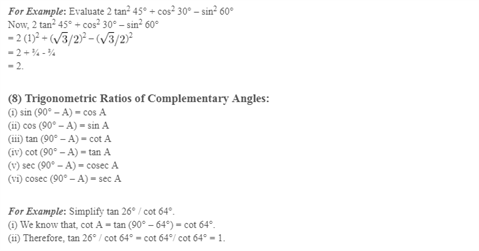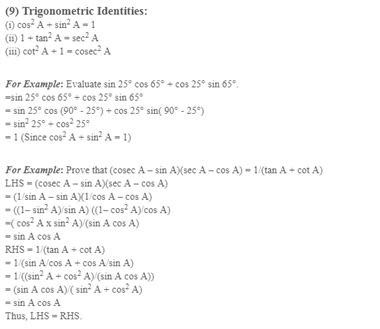Access CBSE Class 10 Maths Sample Papers Here.

Access NCERT Class 10 Maths Book Here.